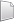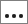# HS-1507: Material Calibration with Area Tool in Data Source

In this tutorial, you will learn how to complete an Area function and create an input template from a Radioss file using the HyperStudy - Editor in HyperStudy.

Before you begin, copy the model files used in this tutorial from <hst.zip>/HS-1507/ to your working directory.

The objective of this tutorial is to find the Radioss material parameter values so that the stress-strain curve of the tensile test simulation matches the tensile test experimental curve.

HS-1506: Material Calibration with a Curve Difference Integral provides an alternative method to setup this problem using a Compose or Python function to measure the difference between two curves.

HS-4200: Material Calibration Using System Identification provides an alternative method using system identification.

By the end of this tutorial, you will know how to:
• Create an input template from a Radioss file using the HyperStudy - Editor
• Set up a study
• Run a MIN(f(x)) optimization study

## Model Definition

A quarter of a standard tensile test specimen is modeled using symmetry conditions. A traction is applied to a specimen via an imposed velocity at the left-end.

The units are: mm, ms, g, N, MPa.
The material to be characterized is a 6063 T7 Aluminum. It has an isotropic elasto-plastic behavior which can be reproduced by a Johnson-Cook model without damage (RADIOSS Block Law2), defined as:
In this tutorial, the parameters a, b, n, σmax (maximum stress), and the Young modulus are defined as input variables. The stress-strain curve obtained by the experimental test is shown in Figure 3.
For the simulation results, engineering strains will be obtained by dividing the displacement of node 1 by the reference length (75 mm), and engineering stresses will be obtained by dividing the force in section 1 by its initial surface (10.2 mm2).

## Create Base Input Template

In this step, you can create the base input template in HyperStudy or use the base input template in the study Directory.

1. Start HyperStudy.
2. From the menu bar, click Tools > Editor.
The Editor opens.
3. In the File field, open the TENSILE_TEST_0000.rad file.
4. In the Find area, enter /MAT/PLAS_JOHNS/1 and click.
HyperStudy highlights /MAT/PLAS_JOHNS/1.
5. Select variable E by highlighting the first 20 fields in row 51.
Tip: Quickly highlight 20-character fields by pressing Ctrl to activate the Selector (set to 20 characters) and then clicking the value.
6. Right-click on the highlighted fields and select Create Parameter from the context menu.
7. In the Parameter: varname_1 dialog, define the following options and click OK.
1. In the Label field, enter E_Young.
2. Change the Lower Bound to 50000.
3. Change the Nominal value to 60400.
4. Change the Upper Bound to 70000.
5. Change the Format to %20.5f.
8. Define four more variables using the information provided in Table 1.
Table 1.
Variable Label Lower Bound Nominal Upper Bound Format
a a_PlasticityYieldStress 90 110 120 %20.5f
b b_HardeningCoeff 100 125 160 %20.5f
n n_HardeningExpo 0.1 0.2 0.3 %20.5f
sigmax Sigma_Max 250 280 290 %20.5f
9. Click Save.
10. In the Save Template dialog, save the file as TENSILE_TEST_0000.tpl.
11. Close the Editor.

## Perform the Study Setup

1. Start a new study in the following ways:
• From the menu bar, click File > New.
• On the ribbon, click.
2. In the Add Study dialog, enter a study name, select a location for the study, and click OK.
3. Go to the Define Models step.
4. Add a Parameterized File model.
1. From the Directory, drag-and-drop the TENSILE_TEST_0000.tpl file into the work area.
2. In the Solver input file column, enter TENSILE_TEST_0000.rad.
This is the name of the solver input file HyperStudy writes during any evaluation.
4. Verify that the Solver input arguments field reads \$file.
This solver input argument runs the Starter and the Engine of RADIOSS for the crash analysis, and also prevents the creation of the .h3d result file from animation files.
Note: X is the number of CPUs to use for the simulation.
5. Define a model dependency.
1. Click Model Resources.
2. In the Model Resource dialog, select Model 1 (m_1).
3. Click Resource Assistant > Add File.
4. In the Select File dialog, navigate to your working directory and open the TENSILE_TEST_0001.rad file.
5. Set Operation to Copy.
6. Click Close.
6. Click Import Variables.
Five input variables are imported from the TENSILE_TEST_0000.tpl resource file.
7. Go to the Define Input Variables step.
8. Review the input variable's lower and upper bound ranges.

## Perform Nominal Run

1. Go to the Test Models step.
2. Click Run Definition.
An approaches/setup_1-def/ directory is created inside the study Directory. The approaches/setup_1-def/run__00001/m_1 directory contains the input file, which is the result of the nominal run.

## Create and Evaluate Output Responses

In this step, you will create the data sources to be used in the Area function and evaluate output responses.

1. Create the Area Between Two Curves output response.
1. Go to the Definition > Define Output Responses step.
3. In the work area, Label field, enter Area Between Two Curves.
2. Create a data source labeled Disp_sim.
1. From the Directory, drag-and-drop the TENSILE_TEST01 file, located in approaches/setup_1-def/run__00001/m_1, into the work area.
2. In the File Assistant dialog, set the Reading technology to Altair® HyperWorks® and click Next.
3. Select Single Item in a Time Series, then click Next.
4. Define the following options, then click Next.
• Set Type to Node/Node 1.
• Set Request to 4 Node 1.
• Set Component to DX-X Displacement.
5. Under Create a new Data Source, enter Disp_sim in the Label field.
6. Clear the Use Data Source in a new Response checkbox.
7. Click Finish.
3. Create a second data source labeled Force_sim by repeating step 2.
Define the following options:
1. Set Type to Section/SECTION_2.
2. Set Request to 2 section 1.
3. Set Component to FT-Resultant Tangent Force.
4. Create a third data source labeled Strain_exp.
1. In the Expression column of the Area Between Two Curves output response, click.
2. In the Expression Builder, click the Data Sources tab.
A new data source is added to the work area.
4. In the Label field for the new data source, enter Strain_exp.
5. In the File field, click.
6. In the Data Sources Builder, define the data source and click OK.
• In the File field, navigate to your working directory and open the experiment.xy file.
• Set Tool to File Source.
• Set Type to Unknown.
• Set Request to Block 1.
• Set Component to Column 1.
5. Create a fourth file source labeled Stress_exp by repeating step 4.
Select the following options:
1. Set Tool to File Source.
2. Set Type to Unknown.
3. Set Request to Block 1.
4. Set Component to Column 2.
6. Define the Area Between Two Curves output response.
1. In the Expression Builder, click the Data Sources tab.
2. Click Add Data Source and choose Area from the Tool options.
3. In the Label field for the new data source, enter Area Difference.
4. In the File column, click.

In the Data Source Builder, there are Curve 1 and Curve 2 options for simulation and experimental curve data. Each has x and y data sources entered differently.

Curve 1_x = Disp_sim

Curve 1_y = Force_sim

Curve 2_x = Strain_exp

Curve 2_y = Stress_exp

The displacements and forces are read from the simulation, whereas from the experiment you have strains and stresses. In order to convert the displacement and forces to strains and stresses, you need to divide the displacements by the length (75) and forces by the area (10.2). Use the scale factor box to define conversions. For displacement, the scale factor is $\frac{1}{75}$. For the area, the scale factor is $\frac{1}{10.2}$.

5. For Curve 1, enter 0.0133333 in the scale factor box for x as shown in Figure 13.
6. For Curve 1, enter 0.0980392 in the scale factor box for y as shown in Figure 13.
7. Click OK to close the Data Source Builder.
7. In the Expression Builder, click Insert Variable.
8. Click OK to close the Expression Builder.
9. Click Evaluate to extract the response values.

## Run Optimization

1. In the Explorer, right-click and select Add from the context menu.
2. In the Add dialog, select Optimization and click OK.
2. Go to the Optimization > Definition > Define Output Responses step.
3. Click the Objectives/Constraints - Goals tab.
4. Apply an objective on the Area Between Two Curves output response.